• 使用动态规划算法需要满足的必要条件：优化原则
2021-11-01 11:11:35

## 1、动态规划的定义

动态规划算法：多阶段决策过程，每步求解的问题是后面阶段求解问题的子问题，每步决策将依赖于以前步骤的决策结果；

## 2、使用动态规划技术的必要条件：满足优化原则

优化原则： 一个最优决策序列的任何子序列本身一定的是相对于子序列的初始和结束状态的最优决策序列；

最优解不满足优化原则的问题不能使用动态规划算法；

## 3、动态规划算法的要素

1. 划分子问题，确定子问题边界，将问题求解转变成多步判断的过程；
2. 定义优化函数，以该函数极大(或极小)值作为依据，确定是否满足优化原则；
3. 列优化函数的递推方程和边界条件；
4. 自底向上计算，设计备忘录（记录每一次计算的值）；
5. 考虑是否需要设立 标记函数（记录每个子问题的解）；
6. 用递归方程或备忘录估计时间复杂度；

见《算法分析与设计》第2版 - 屈婉玲；
见 配套视频教程：https://www.bilibili.com/video/BV1Ls411W7PBleetcode
更多相关内容
• 动态规划算法优化技巧，使用动态规划方法解题，对于不少问题具有空间耗费大、时间效率高的特点，因此人们在研究动态规划解题时更多的注意空间复杂度的优化，运用各种技巧将空间需求控制在软硬件可以承受的范围之内...
• 基于nedc工况的动态规划算法对汽车换档规律进行优化 代码可以在matlab中正常运行 价值非常高，不懂的可以留言学习
• 为了应对随机动态规划算法在解决梯级水电站群长期 发电优化调度时的 “维数灾”问题，并行化方法得到了广泛研究。单机多核并行算法扩展性不强；传统的分布式并行 算法编程复杂，缺少负载均衡和容错机制。云计算平台...
• 63 4.2 贪心算法的基本要素 贪心算法和动态规划算法都要求问题具有最... 下面研究 2 个经典的 组合 优化问题 并以此说明贪心算法与动态规划算法的主要差别 3 贪心算法与动态规划算法的差异 64 4.2 贪心算法的基本要素 ?
• 目录1动态规划思想2适用场景3例题分析3.1示例1：42.接雨水 1动态规划思想 2适用场景 3例题分析 3.1示例1：42.接雨水 题目描述 给定 n 个非负整数表示每个宽度为 1 的柱子的高度图，计算按此排列的柱子，下雨之后能接...

# 1动态规划思想

动态规划算法与分治法类似，其基本思想也是将待求解问题分解成若干个子问题。
动态规划的过程是：每次决策依赖于当前状态，又随即引起状态的转移。一个决策序列就是在变化的状态中产生出来的，所以，这种多阶段最优化决策解决问题的过程就称为动态规划。

# 2适用场景

动态规划常常适用于有重叠子问题和最优子结构性质的问题，动态规划方法所耗时间往往远少于朴素解法。

• 最优化原理：假设问题的最优解所包括的子问题的解也是最优的，就称该问题具有最优子结构，即满足最优化原理。
• 无后效性：即某阶段状态一旦确定。就不受这个状态以后决策的影响。也就是说，某状态以后的过程不会影响曾经的状态。仅仅与当前状态有关。
• 有重叠子问题：即子问题之间是不独立的，一个子问题在下一阶段决策中可能被多次使用到（该性质并非动态规划适用的必要条件，可是假设没有这条性质。动态规划算法同其它算法相比就不具备优势）。

# 3动态规划的三大基本要素

动态规划简单来说就是，利用历史记录，来避免我们的重复计算。而这些历史记录，我们得需要一些变量来保存，一般是用一维数组或者二维数组来保存。下面我们先来讲下做动态规划题很重要的三大基本要素：

• 确定状态和保存状态变量
将问题发展到各个阶段时所处于的各种客观情况用不同的状态表示出来。最简单的就是用数组来保存当前的每一个状态，这个状态就是每个子问题的决策。
• 确定决策并写出状态转移方程
因为决策和状态转移有着天然的联系，状态转移就是根据上一阶段的状态和决策来导出本阶段的状态。所以如果确定了决策，状态转移方程也就可写出。但事实上常常是反过来做，根据相邻两个阶段的状态之间的关系来确定决策方法和状态转移方程。
• 确定边界条件
确定边界条件其实就是跟递归的终止条件是类似的。给出的状态转移方程是一个递推式，需要一个递推的终止条件或边界条件。

# 4解题步骤

一般，只要解决问题的阶段、状态和状态转移决策确定了，就可以写出状态转移方程（包括边界条件）。
根据动态规划的三大基本要素可以设计解题步骤如下：

• 状态定义： 每个状态的决策，存放每个状态的变量，
• 状态转移方程： 当前状态与上一个状态之间的关系
• 初始状态： 初始的状态或者边界条件

# 5例题分析

## 5.1斐波拉契数列

斐波那契数列（Fibonacci sequence），又称黄金分割数列、因数学家莱昂纳多·斐波那契（Leonardoda Fibonacci）以兔子繁殖为例子而引入，故又称为“兔子数列”，指的是这样一个数列：0、1、1、2、3、5、8、13、21、34、……
斐波那契数列以如下被以递推的方法定义：
F ( 1 ) = 1 ， F ( 2 ) = 1 , F ( n ) = F ( n − 1 ) + F ( n − 2 ) （ n ≥ 3 ， n ∈ N ∗ ） F(1)=1，F(2)=1, F(n)=F(n - 1)+F(n - 2)（n ≥ 3，n ∈ N^*）

### 5.1.1递归法求解

由上篇文章递归算法递归算法详解——递归算法的三要素以及例题分析
.
可以写出递归形式的求解为

class Solution {
private final int model = 1000000007;
public int fib(int n) {
if (n < 2){
return n;
}
return ((fib(n - 1) % model + fib(n - 2) % model )) % model;
}
}


答案需要取模 1e9+7（1000000007），如计算初始结果为：1000000008，请返回 1。防止溢出。

若用递归法提交答案后可以看出会超出时间限制。分析可以看出，在递归时会重复计算，如下所示，以F(6)为例：复杂度分析

• 时间复杂度分析： O ( n ) O(n) .。最大递归次数是 n n
• 空间复杂度分析： O ( 1 ) O(1) 。使用常数大小的额外空间。

### 5.1.2动态规划求解

• 状态定义： d p dp 为一维数组，其中 d p [ i ] dp[i] 的值代表 斐波那契数列第 i i 个数字 。
• 转移方程： d p [ i + 1 ] = d p [ i ] + d p [ i − 1 ] dp[i + 1] = dp[i] + dp[i - 1] ，即对应数列定义 f ( n + 1 ) = f ( n ) + f ( n − 1 ) f(n + 1) = f(n) + f(n - 1)
• 初始状态： d p [ 0 ] = 0 , d p [ 1 ] = 1 dp=0, dp = 1 ，即初始化前两个数字； 返回值： d p [ n ] dp[n] ，即斐波那契数列的第 n n 个数字。

空间复杂度优化
若新建长度为 n n d p dp 列表，则空间复杂度为 O ( N ) O(N)

由于 d p dp 列表第 i i 项只与第 i − 1 i−1 和第 i − 2 i-2 项有关，因此只需要初始化三个整形变量 s u m , a , b sum, a, b ，利用辅助变量 s u m sum 使 a , b a, b 两数字交替前进即可 （具体实现见代码） 。
节省了 d p dp 列表空间，因此空间复杂度降至 O ( 1 ) O(1)

class Solution {
public int fib(int n) {
int a = 0, b = 1, sum;
for(int i = 0; i < n; i++){
sum = (a + b) % 1000000007;
a = b;
b = sum;
}
return a;
}
}


复杂度分析

• 时间复杂度分析： O ( n ) O(n) .。最大循环次数是 n n
• 空间复杂度分析： O ( 1 ) O(1) 。使用常数大小的额外空间。

## 5.2剑指offer 42 连续子数组的最大和

题目描述
输入一个整型数组，数组中的一个或连续多个整数组成一个子数组。求所有子数组的和的最大值。

要求时间复杂度为 O ( n ) O(n)
示例1:

输入: nums = [-2,1,-3,4,-1,2,1,-5,4]
输出: 6
解释: 连续子数组 [4,-1,2,1] 的和最大，为 6。

动态规划解析：

• 状态定义： 设动态规划列表 d p dp d p [ i ] dp[i] 代表以元素 n u m s [ i ] nums[i] 为结尾的连续子数组最大和。
\qquad 为何定义最大和 d p [ i ] dp[i] 中必须包含元素 n u m s [ i ] nums[i] ：保证 d p [ i ] dp[i] 递推到 d p [ i + 1 ] dp[i+1] 的正确性；如果不包含 n u m s [ i ] nums[i] ，递推时则不满足题目的 连续子数组 要求。

• 转移方程： d p [ i − 1 ] ≤ 0 dp[i-1] \leq 0 ，说明 d p [ i − 1 ] dp[i - 1] d p [ i ] dp[i] 产生负贡献，即 d p [ i − 1 ] + n u m s [ i ] dp[i-1] + nums[i] 还不如 n u m s [ i ] nums[i] 本身大。
\qquad d p [ i − 1 ] > 0 dp[i - 1] > 0 时：执行$dp[i] = dp[i-1] + nums[i]$ ；
\qquad d p [ i − 1 ] ≤ 0 dp[i - 1] \leq 0 时：执行 d p [ i ] = n u m s [ i ] dp[i] = nums[i]

• 初始状态： d p [ 0 ] = n u m s [ 0 ] dp = nums ，即以 n u m s [ 0 ] nums 结尾的连续子数组最大和为 n u m s [ 0 ] nums

• 返回值： 返回 d p dp 列表中的最大值，代表全局最大值。

class Solution {
public int maxSubArray(int[] nums) {
int res = nums;
for(int i = 1; i < nums.length; i++) {
nums[i] += Math.max(nums[i - 1], 0);
res = Math.max(res, nums[i]);
}
return res;
}
}


复杂度分析

• 时间复杂度 O ( N ) O(N) ： 线性遍历数组 n u m s nums 即可获得结果，使用 O ( N ) O(N) 时间。
• 空间复杂度 O ( 1 ) O(1) ： 使用常数大小的额外空间。

## 3.1示例1：42.接雨水

题目描述
给定 n 个非负整数表示每个宽度为 1 的柱子的高度图，计算按此排列的柱子，下雨之后能接多少雨水。上面是由数组 [0,1,0,2,1,0,1,3,2,1,2,1] 表示的高度图，在这种情况下，可以接 6 个单位的雨水（蓝色部分表示雨水）。
输入输出描述
输入: [0,1,0,2,1,0,1,3,2,1,2,1]
输出: 6
图解模型直观思想

暴力方法中，我们仅仅为了找到最大值每次都要向左和向右扫描一次。但是我们可以提前存储这个值。因此，可以通过动态编程解决。
算法流程

• 找到数组从下标 i i 到最左端最高的条形块高度left_max
• 找到数组从下表 i i 到最右端最高的条形块高度right_max
• 扫描数组height并更新答案：

代码实现

    public int trap(int[] height) {
int len = height.length;
if (len < 2) return 0;
int ans = 0;
int[] max_left = new int[len];
int[] max_right = new int[len];
max_left = height;
for (int i = 1; i < len; i++) {
max_left[i] = Math.max(height[i], max_left[i - 1]);
}
max_right[len - 1] = height[len - 1];
for (int i = len - 2; i >= 0; i--) {
max_right[i] = Math.max(height[i], max_right[i + 1]);
}
for (int i = 1; i < len - 1; i++) {
ans += Math.min(max_right[i], max_left[i]) - height[i];
}
return ans;
}


复杂度分析：

• 时间复杂度： O ( n ) O(n) 。储存最大高度数组需要两次遍历，计算出ans结果遍历一次。
• 空间复杂度： O ( n ) O(n) 。使用2个n数组存放left_max和right_max数组。
展开全文数据结构 java
• m排n列的柱桩，每个柱桩上预先放好价值不一样的宝石。现在有位杂技演员从第一排的第1号柱桩开始跳跃，每次都必须跳到下一排的柱桩上，且每次跳跃最多只能向左或向右移动一个桩子。也就是说如果现在杂技演员站在第j号...
• 在分析最优解结构的基础上,用Java语言给出...全文分为四个部分,首先讨论 了动态规划时间效率优化的可行性和必要性,接着给出了动态规划时间复杂度的决定因素,然后分别阐述了对各个决定因 素的优化方法,最后总结全文。
• ## 详解动态规划算法

千次阅读 多人点赞 2020-04-09 07:59:47
不过动态规划对初学者来说确实比较难，dp状态，状态转移方程让人摸不着头脑，网上很多人也反馈不太好学，其实就像我们之前学递归那样，任何算法的学习都是有它的规律和套路的，只要掌握好它的规律及解题的套路，再...

### 摘要

动态规划（dynamic programming，简称dp)是工程中非常重要的解决问题的思想，从我们在工程中地图软件上应用的最短路径问题，再在生活中的在淘宝上如何凑单以便利用满减券来最大程度地达到我们合理薅羊毛的目的，很多时候都能看到它的身影。不过动态规划对初学者来说确实比较难，dp状态，状态转移方程让人摸不着头脑，网上很多人也反馈不太好学，其实就像我们之前学递归那样，任何算法的学习都是有它的规律和套路的，只要掌握好它的规律及解题的套路，再加上大量的习题练习，相信掌握它不是什么难事，本文将会用比较浅显易懂地讲解来帮助大家掌握动态规划这一在工程中非常重要的思想，相信看完后，动态规划的解题套路一定能手到擒来（文章有点长，建议先收藏再看，看完后一定会对动态规划的认知上升到一个台阶！）

本文将会从以下角度来讲解动态规划：

• 什么是动态规划
• 动态规划从入门到进阶
• 再谈动态规划

### 什么是动态规划

以下是我综合了动态规划的特点给出的动态规划的定义：动态规划是一种多阶段决策最优解模型，一般用来求最值问题，多数情况下它可以采用自下而上的递推方式来得出每个子问题的最优解（即最优子结构），进而自然而然地得出依赖子问题的原问题的最优解。

划重点：

• 多阶段决策，意味着问题可以分解成子问题，子子问题，。。。，也就是说问题可以拆分成多个子问题进行求解
• 最优子结构，在自下而上的递推过程中，我们求得的每个子问题一定是全局最优解，既然它分解的子问题是全局最优解，那么依赖于它们解的原问题自然也是全局最优解。
• 自下而上，怎样才能自下而上的求出每个子问题的最优解呢，可以肯定子问题之间是有一定联系的，即迭代递推公式，也叫「状态转移方程」，要定义好这个状态转移方程， 我们就需要定义好每个子问题的状态（DP 状态），那为啥要自下而上地求解呢，因为如果采用像递归这样自顶向下的求解方式，子问题之间可能存在大量的重叠，大量地重叠子问题意味着大量地重复计算，这样时间复杂度很可能呈指数级上升（在下文中我们会看到多个这样重复的计算导致的指数级的时间复杂度），所以自下而上的求解方式可以消除重叠子问题。
简单总结一下，最优子结构，状态转移方程，重叠子问题就是动态规划的三要素，这其中定义子问题的状态与写出状态转移方程是解决动态规划最为关键的步骤，状态转移方程如果定义好了，解决动态规划就基本不是问题了。

既然我们知道动态规划的基本概念及特征，那么怎么判断题目是否可以用动态规划求解呢，其实也很简单，当问题的定义是求最值问题，且问题可以采用递归的方式，并且递归的过程中有大量重复子问题的时候，基本可以断定问题可以用动态规划求解，于是我们得出了求解动态规划基本思路如下（解题四步曲）

判断是否可用递归来解，可以的话进入步骤 2

• 分析在递归的过程中是否存在大量的重复子问题
• 采用备忘录的方式来存子问题的解以避免大量的重复计算（剪枝）
• 改用自底向上的方式来递推，即动态规划

接下来我们会做几道习题来强化一下大家对这些概念及动态规划解题四步曲的理解，每道题我们都会分别用递归，递归+备忘录，动态规划来求解一遍；

### 动态规划从入门到进阶

#### 入门题：斐波那契数列

接下来我们来看看怎么用动态规划解题四步曲来解斐波那契数列

画外音：斐波那契数列并不是严格意义上的动态规划，因为它不涉及到求最值，用这个例子旨在说明重叠子问题与状态转移方程

1、判断是否可用递归来解 显然是可以的，递归代码如下

public static int fibonacci(int n) {
if (n == 1) return1;
if (n == 2) return2;
return fibonacci(n - 1) + fibonacci(n - 2);
}


2、分析在递归的过程中是否存在大量的重复子问题

怎么分析是否有重复子问题，画出递归树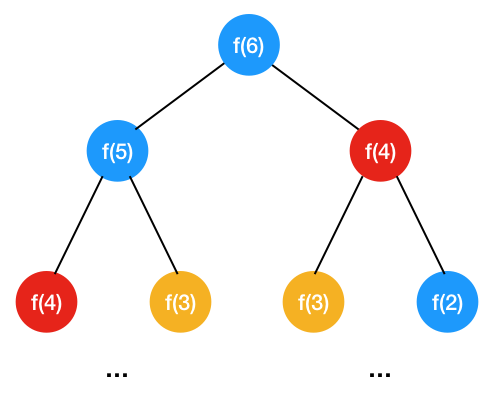可以看到光是求 f(6)，就有两次重复的计算， f(4) 求解了两次，f(3) 求解了两次，时间复杂度是指数级别，递归时间复杂度怎么看，解决每个子问题需要的时间乘以子问题总数，每个子问题需要的时间即 f(n) = f(n-1) + f(n-2) 只做了一次加法运算，子问题的个数有多少呢，每个问题一分为二,是个二叉树，可以看到第一层 1 个，第二层 2 个，第三层 4 个，即 1 + 2 + 2^2 + … 2n，所以总的来说时间复杂度是)O(2n),是指数级别。
画外音：求解问题 f(6),转成求 f(5),f(4),从原问题出发，分解成求子问题，子问题再分解成子子问题，。。。，直到再也不能分解，这种从 原问题展开子问题进行求解的方式叫自顶向下
3、采用备忘录的方式来存子问题的解以避免大量的重复计算 既然以上中间子问题中存在着大量的重复计算，那么我们可以把这些中间结果给缓存住（可以用哈希表缓存），如下

public static int fibonacci(int n) {
if (n = 1) return1;
if (n = 2) return2;
if (map.get(n) != null)  {
return map.get(n);
}
int result = fibonacci(n - 1) + fibonacci(n - 2);
map.put(n, result);
return result;
}


这么缓存之后再看我们的递归树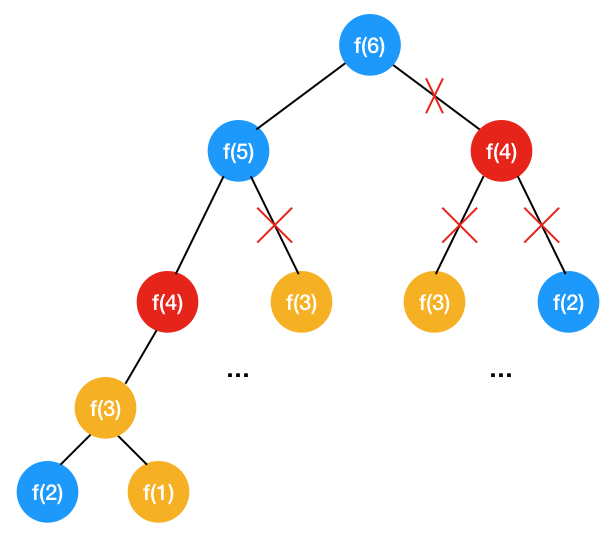可以看到通过缓存中间的数据，做了大量地剪枝的工作，同样的f(4),f(3),f(2)，都只算一遍了,省去了大量的重复计算，问题的规模从二叉树变成了单链表（即 n），时间复杂度变成了 O(n)，不过由于哈希表缓存了所有的子问题的结果，空间复杂度是 O(n)。
4、改用自底向上的方式来递推，即动态规划 我们注意到如下规律

f(1) = 1
f(2) = 2
f(3) = f(1) + f(2) = 3
f(4) = f(3) + f(2) = 5
....
f(n) = f(n-1) + f(n-2)


所以只要依次自底向上求出 f(3),f(4),…,自然而然地就求出了 f(n)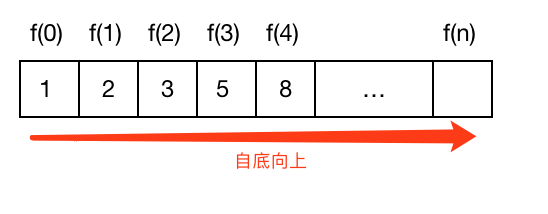画外音：从最终地不能再分解的子问题根据递推方程（f(n) = f(n-1) + f(n-2)）逐渐求它上层的问题，上上层问题，最终求得一开始的问题，这种求解问题的方式就叫自底向上。

f(n) 就是定义的每个子问题的状态（DP 状态），f(n) = f(n-1) + f(n-2) 就是状态转移方程，即 f(n) 由 f(n-1), f(n-2) 这两个状态转移而来,由于每个子问题只与它前面的两个状态，所以我们只要定义三个变量，自底向上不断循环迭代即可，如下

public int f(int n) {
if (n == 1) return1;
if (n == 2) return2;
int result = 0;
int pre = 1;
int next = 2;

for (int i = 3; i < n + 1; i ++) {
result = pre + next;
pre = next;
next = result;
}
return result;
}


这样时间复杂度虽然还是O(n)，但空间复杂度只由于只定义了三个变量（result,pre,next）所以是常量 O(1)。

通过简单地斐波那契的例子，相信大家对自底向上，DP 状态， DP 转移方程应该有了比较深入地认识，细心的同学一定发现了最优子结构怎么没有，因为前面我们也说了，斐波那契数列并不是严格意义上的动态规划，只是先用这个简单地例子来帮助大家了解一下一些基本的概念。在之后的习题中我们将会见识到真正的动态规划

小试牛刀：三角形的最小路径和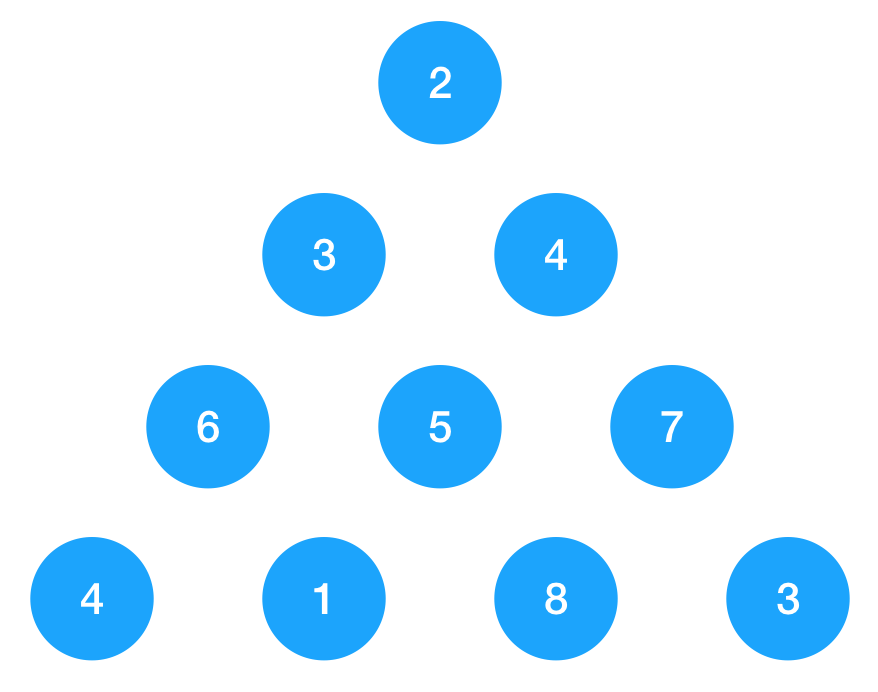如图示，以上三角形由一连串的数字构成，要求从顶点 2 开始走到最底下边的最短路径，每次只能向当前节点下面的两个节点走，如 3 可以向 6 或 5 走，不能直接走到 7。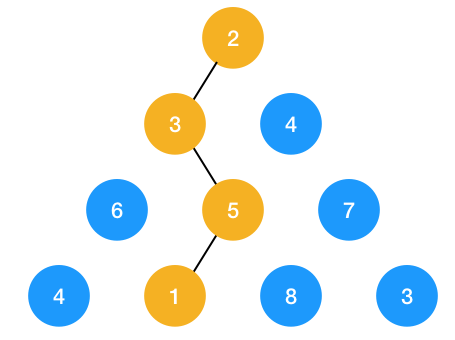如图示:从 2 走到最底下最短路径为 2+3+5+1 = 11,即为我们所求的

首先我们需要用一个二维数组来表示这个三个角形的节点，用二维数组显然可以做到， 第一行的 2 用 a 表示，第二行元素 3, 4 用 a,a,依此类推。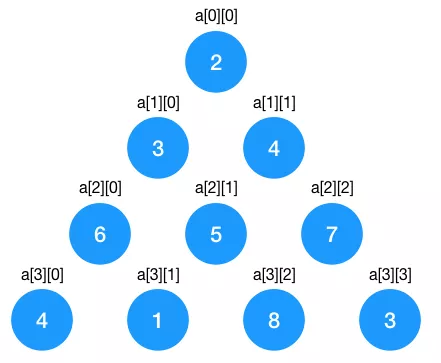定义好数据结构之后，接下来我们来看看如何套用我们的动态规划解题套路来解题

1、 判断是否可用递归来解

如果用递归，就要穷举所有的路径和，最后再求所有路径和的最小值，我们来看看用递归怎么做。

对于每个节点都可以走它的左或右节点，假设我们定义 traverse(i, j) 为节点 a[i][j] 下一步要走的节点，则可以得出递归公式的伪代码如下

traverse(i, j) = {
traverse(i+1, j);    向节点i,j 下面的左节点走一步
traverse(i+1, j+1);    向节点i,j 下面的右节点走一步
}


什么时候终止呢，显然是遍历到三角形最后一条边的节点时终止，发现了吗，对每个节点来说，在往下（无论是往左还是往右）遍历的过程中，问题规模不断地在缩小，也有临界条件（到达最后一条边的节点时终止），分解的子问题也有相同的解决问题的思路（对于每个节点的遍历都是往左或往右），符合递归的条件！于是我们得到递归代码如下

privatestaticint[][] triangle = {
{2, 0, 0, 0},
{3, 4, 0, 0},
{6, 5, 7, 0},
{4, 1, 8, 3}
};

public static int traverse(int i, int j) {
int totalRow = 4; // 总行数
if (i >=  totalRow - 1) {
return0;
}
// 往左下节点走时
int leftSum = traverse(i+1, j) + triangle[i+1][j];
// 往右下节点走时
int rightSum = traverse(i+1, j+1) + triangle[i+1][j+1];
// 记录每个节点往左和往右遍历的路径和的最小值
return Math.min(leftSum, rightSum);
}

public static  void main(String[] args)  throws Throwable {
int sum = traverse(0, 0) + triangle;
System.out.println("sum = " + sum);
}


对于每个节点，要么向左或向右，每个问题都分解成了两个子问题，和斐波那契数列一样，如果画出递归树也是个二叉树，所以时间复杂度是 O(2^n),也是指数级别。

2、分析在递归的过程中是否存在大量的重复子问题

为啥时间复杂度是指数级别呢，我们简单分析一下：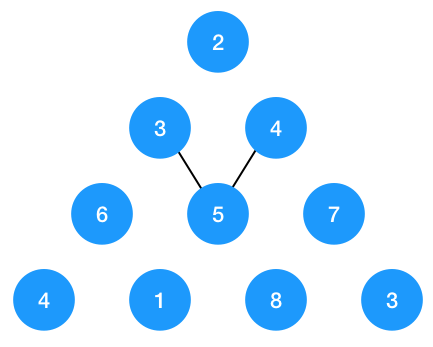对于节点 3 和 4 来说，如果节点 3 往右遍历， 节点 4 往左遍历，都到了节点 5，节点 5 往下遍历的话就会遍历两次，所以此时就会出现重复子问题

3、采用备忘录的方式来存子问题的解以避免大量的重复计算（剪枝）

既然出现了，那我们就用备忘录把中间节点缓存下来

于是我们的代码改为如下所示

privatestaticint[][] triangle = {
{2, 0, 0, 0},
{3, 4, 0, 0},
{6, 5, 7, 0},
{4, 1, 8, 3}
};

// 记录中间状态的 map
privatestatic HashMap<String, Integer> map = new HashMap();

public static int traverse(int i, int j) {
String key = i + "" + j;
if (map.get(key) != null) {
return map.get(key);
}

int totalRow = 4; // 总行数
if (i >=  totalRow - 1) {
return0;
}
// 往左下节点走时
int leftSum = traverse(i+1, j) + triangle[i+1][j];
// 往右下节点走时
int rightSum = traverse(i+1, j+1) + triangle[i+1][j+1];
// 记录每个节点往左和往右遍历的路径和的最小值
int result = Math.min(leftSum, rightSum);
map.put(key, result);
return result;
}


这么一来，就达到了剪枝的目的，避免了重复子问题，时间复杂度一下子下降到 O(n), 空间复杂度呢，由于我们用哈希表存储了所有的节点的状态，所以空间复杂度是 O(n)。

4、改用自底向上的方式来递推，即动态规划

重点来了，如何采用自底向上的动态规划来解决问题呢? 我们这么来看，要求节点 2 到底部边的最短路径，只要先求得节点 3 和 节点 4 到底部的最短路径值，然后取这两者之中的最小值再加 2 不就是从 2 到底部的最短路径了吗，同理，要求节点 3 或 节点 4 到底部的最小值，只要求它们的左右节点到底部的最短路径再取两者的最小值再加节点本身的值（3 或 4）即可。

我们知道对于三角形的最后一层节点，它们到底部的最短路径就是其本身，于是问题转化为了已知最后一层节点的最小值怎么求倒数第二层到最开始的节点到底部的最小值了。先看倒数第二层到底部的最短路径怎么求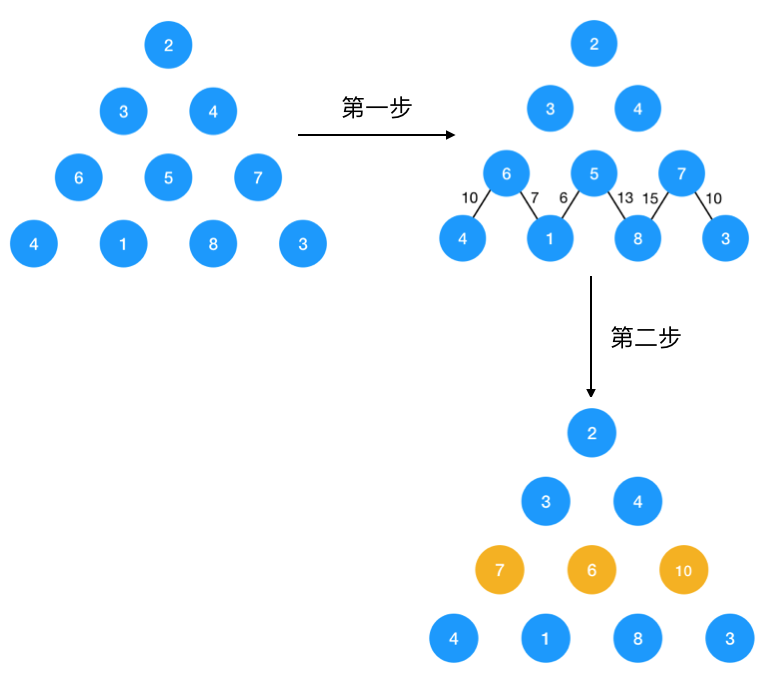同理，第二层对于节点 3 ，它到最底层的最短路径转化为了 3 到 7， 6 节点的最短路径的最小值，即 9, 对于节点 4，它到最底层的最短路径转化为了 4 到 6， 10 的最短路径两者的最小值，即 10。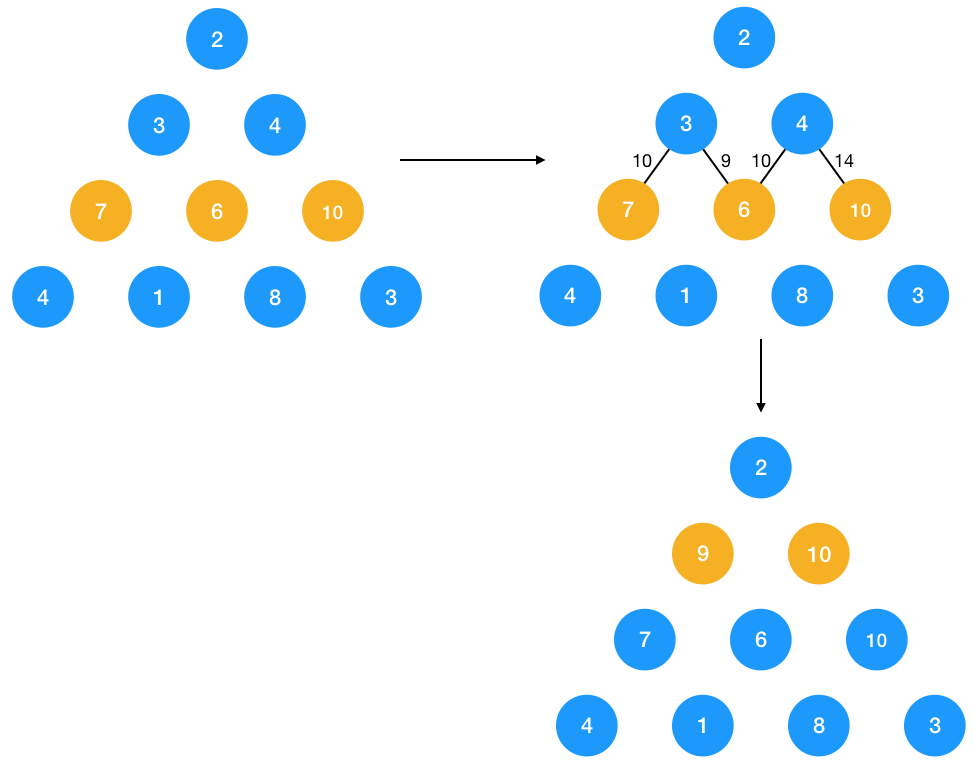接下来要求 2 到底部的路径就很简单了，只要求 2 到节点 9 与 10 的最短路径即可，显然为 11。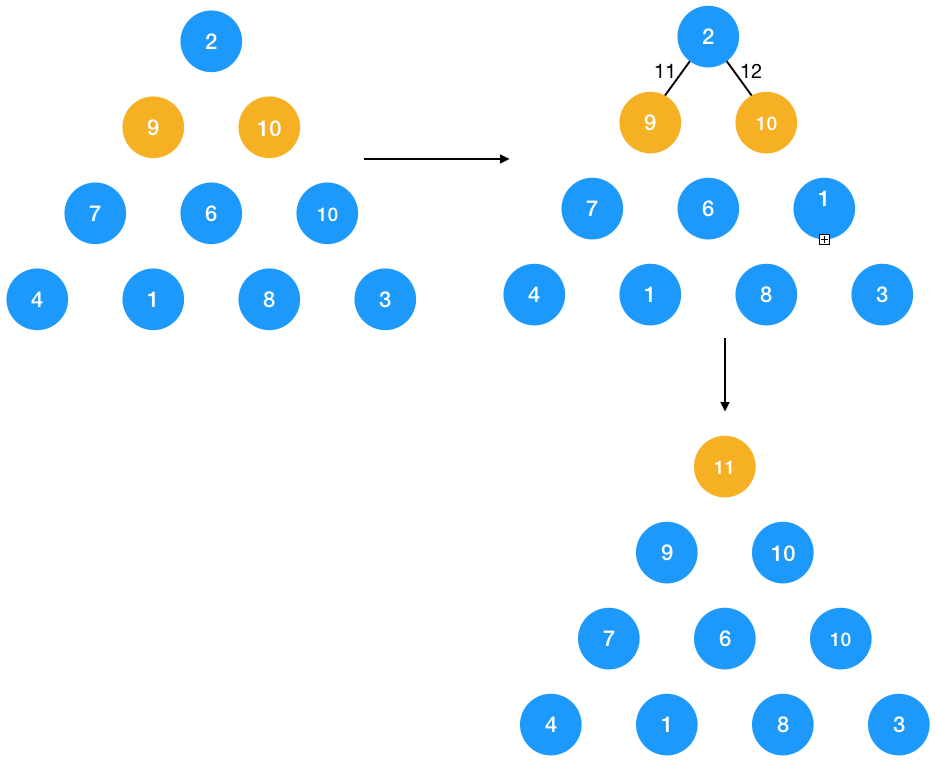于是最终的 11 即为我们所求的值，接下来我们来看看怎么定义 DP 的状态与状态转移方程。我们要求每个节点到底部的最短路径，于是 DP 状态 DP[i,j] 定义为 i,j 的节点到底部的最小值，DP状态转移方程定义如下：

DP[i,j] = min(DP[i+1, j], D[i+1, j+1]) + triangle[i,j]


这个状态转移方程代表要求节点到最底部节点的最短路径只需要求左右两个节点到最底部的最短路径两者的最小值再加此节点本身！仔细想想我们上面的推导过程是不是都是按这个状态转移方程推导的，实在不明白建议多看几遍上面的推导过程，相信不难明白。

DP 状态 DP[i,j] 有两个变量，需要分别从下而上，从左到右循环求出所有的 i,j, 有了状态转移方程求出代码就比较简单了，如下

privatestaticint[][] triangle = {
{2, 0, 0, 0},
{3, 4, 0, 0},
{6, 5, 7, 0},
{4, 1, 8, 3}
};
public static int traverse() {
int ROW = 4;
int[] mini = triangle[ROW - 1];
// 从倒数第二行求起，因为最后一行的值本身是固定的
for (int i = ROW - 2; i >= 0; i--) {
for (int j = 0; j < triangle[j].length; j++) {
mini[j] = triangle[i][j] + Math.min(mini[j], mini[j+1]);
}
}
return mini;
}

public static  void main(String[] args)  throws Throwable {
int minPathSum = traverse();
System.out.println("sum = " + minPathSum);
}


可能有一些人对 mini 数组的定义有疑问，这里其实用了一个比较取巧的方式，首先我们定义 mini 的初始值为最后一行的节点，因为最后一行的每个节点的 DP[i,j] 是固定值，只要从倒数第二行求起即可，其次我们知道每个节点到底部的最短路径只与它下一层的 D[I+1,j], D[i+1, j] 有关，所以只要把每一层节点的 DP[i,j] 求出来保存到一个数组里即可，就是为啥我们只需要定义一下 mini 一维数组的原因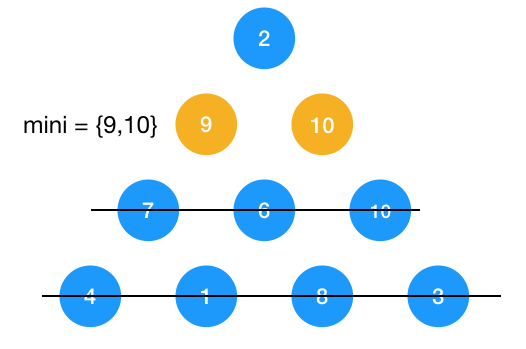如图示：要求节点 2 到底部的最短路径，它只关心节点 9， 10，之前层数的节点无需再关心！因为 9，10 已经是最优子结构了，所以只保存每层节点（即一维数组）的最值即可！

当自下而上遍历完成了，mini 的值即为 DP[0,0],即为节点 2 到 底部的最短路径。mini 的定义可能有点绕，大家可以多思考几遍，当然，你也可以定义一个二维数组来保存所有的 DP[i,j]，只不过多耗些空间罢了。

这里我们再来谈谈最优子结构，在以上的推导中我们知道每一层节点到底部的最短路径依赖于它下层的左右节点的最短路径，求得的下层两个节点的最短路径对于依赖于它们的节点来说就是最优子结构，最优子结构对于子问题来说属于全局最优解，这样我们不必去求节点到最底层的所有路径了，只需要依赖于它的最优子结构即可推导出我们所要求的最优解，所以最优子结构有两层含义，一是它是子问题的全局最优解，依赖于它的上层问题只要根据已求得的最优子结构推导求解即可得全局最优解，二是它有缓存的含义，这样就避免了多个依赖于它的问题的重复求解（消除重叠子问题）。

总结：仔细回想一下我们的解题思路，我们先看了本题是否可用递归来解，在递归的过程中发现了有重叠子问题，于是我们又用备忘录来消除递归中的重叠子问题，既然我们发现了此问题可以用递归+备忘录来求解，自然而然地想到它可以用自底向上的动态规划来求解。是的，求解动态规划就按这个套路来即可，最重要的是要找出它的状态转移方程，这需要在自下而上的推导中仔细观察。

#### 进阶：凑零钱

给定不同面额的硬币 coins 和一个总金额 amount。编写一个函数来计算可以凑成总金额所需的最少的硬币个数。如果没有任何一种硬币组合能组成总金额，返回 -1。输入: coins =
[1, 2, 5], amount = 11，输出: 3 解释: 11 = 5 + 5 + 1 输入: coins = ,
amount = 3，输出: -1

来套用一下我们的动态规划解题四步曲

一、判断是否可用递归来解

对于 amount 来说，如果我们选择了 coins 中的任何一枚硬币，则问题的规模(amount)是不是缩小了,再对缩小的 amount 也选择 coins 中的任何一枚硬币,直到再也不能选择（amount <= 0, amount = 0 代表有符合条件的解，小于0代表没有符合条件的解），从描述中我们可以看出问题可以分解成子问题，子问题与原问题具有相同的解决问题的思路，同时也有临界条件，符合递归的条件，由此可证可以用递归求解，接下来我们来看看，如何套用递归四步曲来解题

1、定义这个函数，明确这个函数的功能,函数的功能显然是给定一个 amount，用定义好的 coins 来凑，于是我们定义函数如下

private static int f(int amount, int[] coins) {
}


2、寻找问题与子问题的关系，即递推公式 这题的递推关系比较难推导，我们一起看下，假设 f(amount, coins) 为零钱 amount 的所需要的最少硬币数，当选中了coins 中的第一枚硬币之后（即为 coins），则需再对剩余的 amount - coins 金额求最少硬币数，即调用 f(amount - coins, coins) ,由此可知当选了第一枚硬币后的递推公式如下

f(amount, coins) = f(amount-coins) + 1 (这里的 1 代表选择了第一枚硬币)


如果选择了第二，第三枚呢，递推公式如下

f(amount, coins) = f(amount-coins) + 1 (这里的 1 代表选择了第二枚硬币)
f(amount, coins) = f(amount-coins) + 1 (这里的 1 代表选择了第三枚硬币)


我们的目标是求得所有以上 f(amount, coins) 解的的最小值，于是可以得到我们的总的递推公式如下

f(amount, coins) = min{ f(amount - coins[i]) + 1) }, 其中 i 的取值为 0 到 coins 的大小，coins[i] 表示选择了此硬币, 1 表示选择了coins[i]  这一枚硬币


3、将第二步的递推公式用代码表示出来补充到步骤 1 定义的函数中

得出了递推公式用代码实现就简单了，来简单看一下

publicclass Solution {

private static int exchange(int amount, int[] coins) {

// 说明零钱刚好凑完
if (amount == 0) {
return0;
}

// 说明没有满足的条件
if (amount < 0) {
return -1;
}

int result = Integer.MAX_VALUE;
for (int i = 0; i < coins.length; i++) {
int subMin = exchange(amount - coins[i], coins);
if (subMin == -1) continue;
result = Math.min(subMin + 1, result);
}

// 说明没有符合问题的解
if (result == Integer.MAX_VALUE) {
return -1;
}

return result;
}

public static  void main(String[] args)  throws Throwable {
int amount = 11;
int[] coins = {1,2,5};
int result = exchange(amount, coins);
System.out.println("result = " + result);
}
}


4、计算时间复杂度 这道题的时间复杂度很难看出来，一般看不出来的时候我们可以画递归树来分析，针对 amount = 11 的递归树 如下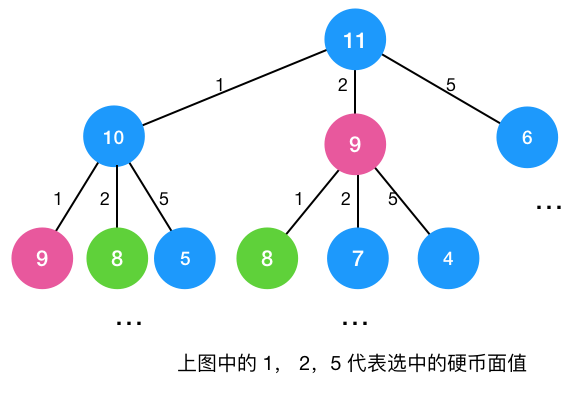前文我们说到斐波那契的递归树是一颗二叉树，时间复杂度是指数级别，而凑零钱的递归树是一颗三叉树 ，显然时间复杂度也是指数级别!

二、分析在递归的过程中是否存在大量的重叠子问题（动态规划第二步）

由上一节的递归树可知，存在重叠子问题，上一节中的 9， 8，都重复算了,所以存在重叠子问题！

三、采用备忘录的方式来存子问题的解以避免大量的重复计算（剪枝）

既然我们知道存在重叠子问题，那么就可以用备忘录来存储中间结果达到剪枝的目的

publicclass Solution {

// 保存中间结果
privatestatic HashMap<Integer, Integer> map = new HashMap();

// 带备忘录的递归求解
private static int exchangeRecursive(int amount, int[] coins) {
if (map.get(amount) != null) {
return map.get(amount);
}
// 说明零钱已经凑完
if (amount == 0) {
return0;
}

// 说明没有满足的条件
if (amount < 0) {
return -1;
}

int result = Integer.MAX_VALUE;
for (int i = 0; i < coins.length; i++) {
int subMin = exchangeRecursive(amount - coins[i], coins);
if (subMin == -1) continue;
result = Math.min(subMin + 1, result);
}

// 说明没有符合问题的解
if (result == Integer.MAX_VALUE) {
return -1;
}

map.put(amount, result);
return result;
}

public static  void main(String[] args)  throws Throwable {
int amount = 11;
int[] coins = {1,2,5};
int result = exchangeRecursive(amount, coins);
System.out.println("result = " + result);
}
}


四、改用自底向上的方式来递推，即动态规划

前面我们推导出了如下递归公式

f(amount, coins) = min{ f(amount - coins[i]) + 1) }, 其中 i 的取值为 0 到 coins 的大小，coins[i] 表示选择了此硬币, 1 表示选择了coins[i]  这一枚硬币


从以上的递推公式中我们可以获取 DP 的解题思路，我们定义 DP(i) 为凑够零钱 i 需要的最小值，状态转移方程如下

DP[i] =  min{ DP[ i - coins[j] ] + 1 } = min{ DP[ i - coins[j] ]} + 1,  其中 j 的取值为 0 到 coins 的大小，i 代表取了 coins[j] 这一枚硬币。


于是我们只要自底向上根据以上状态转移方程依次求解 DP, DP,DP.,…DP，最终的 DP，即为我们所求的解

// 动态规划求解
private static int exchangeDP(int amount, int[] coins) {
int[] dp = newint[amount + 1];
// 初始化每个值为 amount+1，这样当最终求得的 dp[amount] 为 amount+1 时，说明问题无解
for (int i = 0; i < amount + 1; i++) {
dp[i] = amount + 1;
}

// 0 硬币本来就没有，所以设置成 0
dp = 0;

for (int i = 0; i < amount + 1; i++) {
for (int j = 0; j < coins.length; j++) {
if (i >= coins[j]) {
dp[i] = Math.min(dp[i- coins[j]], dp[i]) + 1;
}
}
}

if (dp[amount] == amount + 1) {
return -1;
}
return dp[amount];
}


画外音：以上只是求出了凑成零钱的的最小数量，但如果想求由哪些面值的硬币构成的，该如何修改呢？

凑零钱这道题还可以用另外一道经典的青蛙跳台阶的思路来考虑，从最底部最少跳多少步可以跳到第 11 阶，一次可以跳 1，2，5步 。由此可知最后一步一定是跳 1 或 2 或 5 步，于是如果用 f(n) 代表跳台阶 n 的最小跳数，则问题转化为了求 f(n-1)，f(n-2) ，f(n-5)的最小值。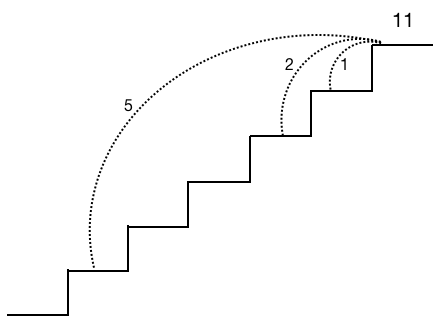如图示：最后一跳一定是跳 1 或 2 或 5 步，只要求 f(n-1)，f(n-2) ，f(n-5)的最小值即可

写出递推表达式， 即：

 f(n) = min{ f(n-1)，f(n-2)，f(n-5)} + 1 （1代表最后一跳）


我们的 DP 状态转移方程对比一下，可以发现两者其实是等价的，只不过这种跳台阶的方式可能更容易理解。

### 总结

本文通过几个简单的例子强化了大家动态规划的三要素：最优子结构，状态转移方程，重叠子问题的理解，相信大家对动态规划的理解应该深刻了许多，怎么看出是否可以用动态规划来解呢，先看题目是否可以用递归来推导，在用递归推导的过程如果发现有大量地重叠子问题，则有两种方式可以优化，一种是递归+ 备忘录，另一种就是采用动态规划了，动态规划一般是自下而上的， 通过状态转移方程自下而上的得出每个子问题的最优解（即最优子结构），最优子结构其实也是穷举了所有的情况得出的最优解，得出每个子问题的最优解后，也就是每个最优解其实是这个子问题的全局最优解，这样依赖于它的上层问题根据状态转移方程自然而然地得出了全局最优解。动态规划自下而上的求解方式还有一个好处就是避免了重叠子问题，因为依赖于子问题的上层问题可能有很多，如果采用自顶而下的方式来求解，就有可能造成大量的重叠子问题，时间复杂度会急剧上升。

展开全文• 此ppt介绍了解决TSP（旅行商问题）的三种算法动态规划、蚁群算法、遗传算法
• 动态规划算法的实质是更好的优化穷举算法，即把算过的子树记录下来减少计算次数。 储存计算过的子树的数列就是dp数列 使用动态规划有三个条件 1.最值问题 一般动态规划的使用场景是在求最值问题中，别的使用场景目前...
• 在求解一维连续型动态规划问题的自创算法—–离散近似迭代法的基础上, 结合双收敛方法, 对多维连续 型动态规划问题进行计算. 该算法的基本思路为: 在给定其他状态向量序列的基础上, 每次对一个状态变量序列进行...
• 算法合集之从鹰蛋一题浅析对动态规划算法优化PPT学习教案.pptx
• 在现代战争中如何利用有限资源对多批来袭目标进行最优排序拦截和资源最佳分配成为一个重要问题，文中采用了一种动态规划算法对不平衡来袭目标进行优化计算，通过建立动态模型，确定状态变量、决策变量以及各个阶段的...
• 动态规划算法时间效率的优化PPT学习教案.pptx
• 在此基础上运用遗传算法获得考虑负荷变化情况下配电网低压侧无功补偿装置的动态最优规划结果。详细讨论了投资规模不加限制、限制总投资规模和限制各个阶段投资规模三种情况的处理方法。对实例的分析表明。提出的方法...
• 最近看缓存相关论文，里面提到动态规划算法来解决小规模组合优化最优解，便尝试复DP算法，论文给出了一个简单例子，先从实现该例子开始，话说动态规划算法可以写好多东西，作为一个外行，第一次接触动态规划，...

### matlab实现动态规划算法

最近看缓存相关论文，里面提到动态规划算法来解决小规模组合优化最优解，便尝试复DP算法，论文给出了一个简单例子，先从实现该例子开始，话说动态规划算法可以写好多东西，作为一个外行，第一次接触动态规划，断断续续花了一周多时间实现该算法，不知道这个效率去公司是不是已经被炒鱿鱼了。动态规划入门简单例子肯定从背包问题说起。
背包问题，讲解的通俗易懂，看懂后可以大致了解动态规划步骤，看懂后这种思想便可以移植到其他问题上。

## 论文例子

论文title《Mobility-Aware Caching in D2D Networks》，发表在IEEE TRANSACTIONS ON WIRELESS COMMUNICATIONS。
两个用户需要缓存文件，文件总数3，用户缓存容量为2，怎样缓存取得最优值。文章给出的DP算法。只要看懂上图的例子，并实现，下面的算法就很好实现了。不同文章DP算法几乎类似。就是一个多阶段决策优化问题。## 实现算法

从例子图可知：stage1阶段，只能缓存文件1，到stage2阶段，就可以缓存文件2，在缓存文件1得到的最优值基础上，加上缓存文件2，可以从Uf表中得到，便可得到新的缓存后的最优值，对每一个state，有不同的组合，这个状态就是可以缓存的最大容量，必须要在容量内进行组合，并比较取得最优值，从stage3，多个stage2的状态到stage3状态（2 2），最后比较得到整个决策过程的最优值。
matlab实现需要准备初始的stage1，和Uf，不断递归，得到最后一个stage，找到最优值及缓存方案。
从图中看，state状态需要矩阵保存，缓存分配需要矩阵保存，那么采用元胞数组来保存每一个stage。Uf也采用元胞保存。递归关系就是前一阶段某一状态的最优值加上Uf里的各种组合的值，最后取最优值并保存最优分配方案。

## 代码

核心部分

for f = 2:F
for i = 1:size(state,1)
for j = 1:size(Uf,1)
a = findAB(state,[sum(cell2mat(stage{1,f-1}(i,2)),1)+cell2mat(Uf(j,1))]);
if a == 0
continue
end
b = cell2mat(stage{1,f-1}(i,3))+cell2mat(Uf(j,f+1));
if cell2mat(stage{1,f}(a,3))<=b
stage{1,f}(a,3)={b};
stage{1,f}(a,2)= {[cell2mat(stage{1,f-1}(i,2));cell2mat(Uf(j,1))]};
end
end
end
end
`

运行后stage3的结果，最优值0.82，对应缓存矩阵[1 1；1 0；0 1]。展开全文matlab
• 针对传统动态规划算法在计算大规模路网的优化问题时所表现出来的计算时间长、存储空间大等缺点,引入了一种神经动态规划算法:它将传统的动态规划和BP神经网络结合起来,通过逼近Q学习算法来寻求一种最优策略,最终达到...
• 针对炼钢组炉计划编制中的集约优化问题，建立了各优化目标下的数学模型，并利用动态规划法，对该优化问题进行了求解。经算法时间复杂性分析和实际生产数据仿真演算，结果表明在一定的生产条件下，该算法能在合理的...
• 然后对本算法进行了时间复杂性和空间复杂性分析，得到时间复杂性由通常的动态规划算法的O(Mn2)提高到本算法的O(n3)，空间复杂性由通常的动态规划算法的O(Mn)提高到本算法的O(n2)，因此效率有了较大提高。最后通过...
• 针对智能交通领域中动态轨迹点的地图匹配问题,提出并设计了一种基于动态规划算法的轨迹匹配软件,并在路网拓扑构建、最短路径计算方面进行改进优化,提升了软件工作性能。工程应用表明,该软件具有较好的计算精度和效率...
• 大数据-算法-中长期发电优化调度的近似动态规划模型与算法.pdf
• 动态规划算法时间效率的优化 动态规划算法时间效率的优化...